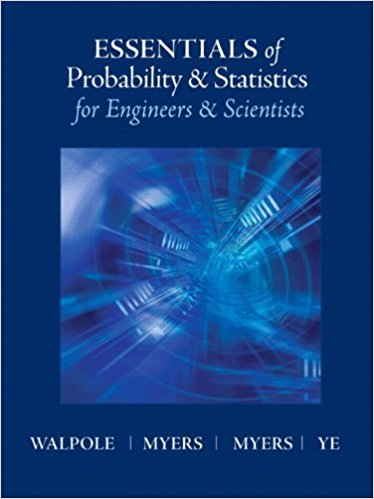×
Log in to StudySoup
Get Full Access to Probability and Statistics - Textbook Survival Guide
Join StudySoup for FREE
Get Full Access to Probability and Statistics - Textbook Survival Guide

Already have an account? Login here
×
Reset your password

# Solutions for Chapter 8: One-Factor Experiments: General## Full solutions for Essentials of Probability & Statistics for Engineers & Scientists | 1st Edition

ISBN: 9780321783738Solutions for Chapter 8: One-Factor Experiments: General

Solutions for Chapter 8
4 5 0 303 Reviews
12
4
##### ISBN: 9780321783738

This expansive textbook survival guide covers the following chapters and their solutions. Essentials of Probability & Statistics for Engineers & Scientists was written by and is associated to the ISBN: 9780321783738. Since 47 problems in chapter 8: One-Factor Experiments: General have been answered, more than 22567 students have viewed full step-by-step solutions from this chapter. Chapter 8: One-Factor Experiments: General includes 47 full step-by-step solutions. This textbook survival guide was created for the textbook: Essentials of Probability & Statistics for Engineers & Scientists, edition: 1.

Key Statistics Terms and definitions covered in this textbook
• 2 k factorial experiment.

A full factorial experiment with k factors and all factors tested at only two levels (settings) each.

• `-error (or `-risk)

In hypothesis testing, an error incurred by rejecting a null hypothesis when it is actually true (also called a type I error).

• Asymptotic relative eficiency (ARE)

Used to compare hypothesis tests. The ARE of one test relative to another is the limiting ratio of the sample sizes necessary to obtain identical error probabilities for the two procedures.

• Backward elimination

A method of variable selection in regression that begins with all of the candidate regressor variables in the model and eliminates the insigniicant regressors one at a time until only signiicant regressors remain

• Bayes’ estimator

An estimator for a parameter obtained from a Bayesian method that uses a prior distribution for the parameter along with the conditional distribution of the data given the parameter to obtain the posterior distribution of the parameter. The estimator is obtained from the posterior distribution.

• Bernoulli trials

Sequences of independent trials with only two outcomes, generally called “success” and “failure,” in which the probability of success remains constant.

• Bimodal distribution.

A distribution with two modes

• Binomial random variable

A discrete random variable that equals the number of successes in a ixed number of Bernoulli trials.

• C chart

An attribute control chart that plots the total number of defects per unit in a subgroup. Similar to a defects-per-unit or U chart.

• Categorical data

Data consisting of counts or observations that can be classiied into categories. The categories may be descriptive.

• Central composite design (CCD)

A second-order response surface design in k variables consisting of a two-level factorial, 2k axial runs, and one or more center points. The two-level factorial portion of a CCD can be a fractional factorial design when k is large. The CCD is the most widely used design for itting a second-order model.

• Control limits

See Control chart.

• Critical region

In hypothesis testing, this is the portion of the sample space of a test statistic that will lead to rejection of the null hypothesis.

• Discrete distribution

A probability distribution for a discrete random variable

• Distribution free method(s)

Any method of inference (hypothesis testing or conidence interval construction) that does not depend on the form of the underlying distribution of the observations. Sometimes called nonparametric method(s).

• Eficiency

A concept in parameter estimation that uses the variances of different estimators; essentially, an estimator is more eficient than another estimator if it has smaller variance. When estimators are biased, the concept requires modiication.

• Erlang random variable

A continuous random variable that is the sum of a ixed number of independent, exponential random variables.

• Error propagation

An analysis of how the variance of the random variable that represents that output of a system depends on the variances of the inputs. A formula exists when the output is a linear function of the inputs and the formula is simpliied if the inputs are assumed to be independent.

• Finite population correction factor

A term in the formula for the variance of a hypergeometric random variable.

• Gamma random variable

A random variable that generalizes an Erlang random variable to noninteger values of the parameter r